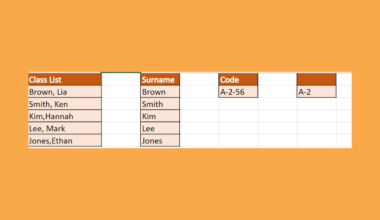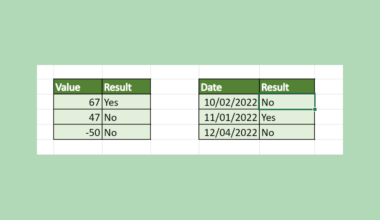# How to Backwards Vlookup in Reverse Order in Excel

This guide will discuss how to backwards VLOOKUP in reverse order in Excel with the help of other functions.

In other words, we need to use other functions to successfully perform backwards `VLOOKUP` in reverse order in Excel, meaning we will search from right to left.

The `VLOOKUP` function is often used to search for specific data from another range or column. So `VLOOKUP` searches for the specified data and pulls the matching data. But it can only search starting from the left to the right.

The `VLOOKUP` function is easy to use. But what if we want to search starting from right to left? Since the `VLOOKUP` function cannot search from right to left, we will utilize other functions to perform this.

So we will use a combination of the `INDEX` and `MATCH` functions. First, the `INDEX` function is for the cell reference. `INDEX` returns the reference to a value or a specific value within a range or table.

While the `MATCH` function works similarly to the `VLOOKUP` function. `MATCH` also searches for the specified data in a range, but it returns the data’s position instead of the data’s actual value.

Let’s take an example.

Suppose you are a teacher who wants to group the students by their birth month. So you are trying to search for all the people who will be having their birthday in August. But the data you are looking up is located on the left of the value column, which is the employee names column.

Instead of using the `VLOOKUP` function, you utilized the `MATCH` function to search for the position of the birthday month. And the `INDEX` function pulls the employee name of that specific position.

Great! Let’s move on and dissect the parts of the `INDEX` and `MATCH` functions.

## The Anatomy of the INDEX Function

The syntax or the way we write the `INDEX` function is as follows:

` =INDEX(array; row_num; [column_num]; [area_num])`

Let’s dissect this formula and understand what each term means:

• = this is how we start any function in Excel
• INDEX() is our `INDEX` function. This function returns a reference of a cell or a value in a given range.
• array refers to the range or array. So this is where you will be searching the position and pulling data or value from.
• row_num is the specific row of the array or reference from which we will pull the data or value.
• column_num refers to the column position of the array or reference. This is an optional argument.
• area_num is the specified range in a reference that we will be using. This is also an optional argument.

## The Anatomy of the MATCH Function

The syntax or the way we write the `MATCH` function is as follows:

` =MATCH(lookup_value; lookup_array; [match_type])`

Let’s take each part and learn what each term means:

• = this equal sign starts every function in Excel.
• MATCH() is our `MATCH` function. It returns the position of a specified value or data in a range or array that matches the specified order.
• lookup_value refers to the value we will use to look for a specific value or data in a range.
• lookup_array is the range where the possible lookup values is located. It can be a reference to a range or array.
• match_type is an optional argument. It refers to the exact or approximate match of what you are searching for. So 1, which is the default, means an exact match or the next smallest value to the specified data, 0 means exact match, and -1 means exact or next largest value to the specified data.

Now let’s dive into a real example of performing backwards `VLOOKUP` in reverse order in Excel.

## A Real Example of Backwards VLOOKUP in Reverse Order in Excel

Let’s take a look at our sample spreadsheet. For instance, we want to look for the students’ names based on their class numbers.So the class number is located on the right of the students’ names. We need to search from right to left. With the combination of the `INDEX` function and the `MATCH` function, we can perform a backwards `VLOOKUP` in reverse order in Excel.

How does it work? First, the `MATCH` function searches for a match of the given class number. Then, it will return the cell reference of the class number that matches what was specified.

For example, we are looking for student B3. So the `MATCH` function will return the cell reference for B3 within the range. Next, the `INDEX` function will return the student’s name from the column we specified based on the given cell reference from the `MATCH` function.

Basically, the `MATCH` function returns 4, which is the cell reference for B3. Then, the `INDEX` function returns Ethan, which is in cell 4 or row 4 in the Student column.Awesome! Now that we have seen a real example and learned how the formula works. Let’s learn the steps on how to backwards `VLOOKUP` in reverse order in Excel.

## How to Backwards VLOOKUP in Reverse Order in Excel

In this section, we will explain the step-by-step process of how to perform backwards `VLOOKUP` in Reverse Order in Excel using the `INDEX` function and the `MATCH` function.

1. First, create a separate table where we can input our search results. In the first column of the table, type the value or data we will use in our search. In this case, we will input the class numbers, which we will use to look for the corresponding student.2. Second, input the formula  “=INDEX(\$B\$2:\$B\$11;MATCH(D2;\$A\$2:\$A\$11;0)”  in the second column or wherever you want to place the returned results. Then, press the Enter key to return the result.For the lookup_value of `MATCH`, we can simply select the class number on the first column for our cell reference. And we input “0” to search for the exact match in the range.

Then, we simply select the column from which we will pull the result for the `INDEX` function. In this case, we want the student’s name. So we select the Student column.

3. Next, drag down to copy the formula to the rest of the column. This will automatically use the formula to look for the students based on the given class number.4. And tada! We have successfully performed `VLOOKUP` from right to left using the `INDEX` and `MATCH` functions.That’s pretty much it! Now you can perform backwards `VLOOKUP` in reverse order in Excel whenever you want to search from right to left.

Are you interested in learning more about what Google Sheets can do? You can now use the `VLOOKUP` function in Google Sheets and the various other Google Sheets formulas available to create great worksheets that work for you. Make sure to subscribe to our newsletter to be the first to know about the latest guides and tutorials from us.Our goal this year is to create lots of rich, bite-sized tutorials for Google Sheets users like you. If you liked this one, you'll love what we are working on! Readers receive ✨ early access ✨ to new content.

##### You May Also Like## How To Calculate A Dot Product In Excel

This guide will discuss how to calculate a dot product in Excel using the SUMPRODUCT function.  The dot…## How to Use the PMT Function in Excel

This guide will explain how to use the PMT function in Excel. The rules for using the PMT…## How to Find the Position of the Nth Space on a String in Excel

The FIND function in Excel is useful when you need to find the position of the nth space…## How to Use TEXTBEFORE Function in Excel

This guide will discuss how to use the TEXTBEFORE function in Excel.  The rules for using the TEXTBEFORE…